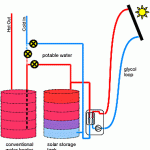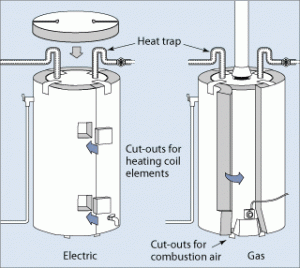# Standing heat losses in a solar water heat systemIn this post we are going to calculate the typical standing losses from both the solar storage tank, and from the conventional electric water heater tank on a solar water heating system, as well as provide some options for minimizing the costs associated with standing losses. �For the solar storage tank, we will estimate the over-night temperature drop associated with the heat loss, where for the conventional water heater, we will determine the associated electrical energy costs required to overcome the standing heat losses.

Although representative temperature and heat loss values have been selected, the exact values will differ between installs, and the numbers in this post are intended to provide a general idea, rather than to detail exact or expected values.

The general equation for heat loss from a water heater is of the form:
H = A (?T)/R
Where Hheat loss in btu
Asurface area of water heater in square feet
?T – delta T, water temperature minus�ambient�air temperature in��F
R - thermal resistance of the tank insulation in ft���F/btu�. �Assume R9.

• Determine A for a standard tank 60″ (152 cm) tall and 22″ (56 cm) diameter.
• side area = height x pi x diameter = 60 x 3.14 x 22 = 4145 in�
• base + top area = 2 x pi x r� = 2 x pi x (diameter/2)� = 2 x 3.14 x (22/2)� = 760 in�
• Total area = 760 in� + 4145 in� = 4905 in� = 34 ft� = 3.1 m�
• Estimate�?T
• Assume�ambient�temperature is 68�F (20�C)
• Assume conventional water heater is 130�F (54�C)
• Assume solar storage tank is 170�F (77�C)
• Conventional�?T = 130 – 68 = 62�F (34�C)
• Solar storage�?T = 170 – 68 = 102�F (57�C)
• Heat loss – Conventional
• H =�A (?T)/R
• H = 34 ft� x 62�F/ 9�ft���F/btu = 234 btu (per hour)
• H = 5621 btu/day (1.64 kWh/day)
• Heat loss – Solar Storage Tank
• H =�A (?T)/R
• H = 34�ft� x 102�F/ 9�ft���F/btu = 385 btu (per hour)
• H = 9248 btu/day (2.71 kWh/day)

What does it mean? �If you are not using any hot water for a day, your conventional electric water heater will lose 1.64 kWh (5621 btu) of energy, or about \$0.20 worth of electricity per day.

For the solar storage tank, there would be about 2.7 kWh per day, or 1/2 of that lost each night. �Since the solar energy is free (Thank you, Mr. Sunshine!) it isn’t directly costing you money, if the following day is sunny, the energy will be replaced the following morning. However, the tank will have cooled off over night, and if the following day(s) are not good solar days, the temperature will continue to drop.

• Calculating solar storage tank�temperature drop (Td)
• Td = H/m
• m – mass of water in the tank in lbs – or 668 lbs for 80 gallons
• H – heat loss from tank in btu – calculated at 9248 btu/day
• Td = 9248/668 = 13.8��F/day (7.7�C/day)
• Or about 1/2 of that value lost over night.

Reducing standing losses – �although the default configuration is satisfactory for most home owners, there are some options available for reduction of standing energy losses. The 1st item listed (increase insulation) is something that can be considered for all water tanks, solar or not, however, extra care must always be taken when attaching any sort of covering to a gas water heater.• The standing losses for both tanks can be reduced by improving the insulation levels of the storage tanks. �If a home owner increased an R9 tank by and additional R18, standing losses would be cut in 1/3. �Here is a good US government article on adding insulation to a water tank.
• Drawing hot water out of the system will cause solar heated water to enter the conventional water heating tank. �When the solar storage temperature is higher than the thermostat temperature of the conventional tank, it will introduce “free” heat into that tank, and reduce the amount of conventional energy required to overcome the standing losses.
• It may be acceptable to turn off the conventional storage tank elements, allowing the average temperature to drop a bit, but eliminating electrical consumption to overcome standing losses.
• In some cases, the two tanks can be plumbed “in parallel” which allows solar to heat both tanks, and the conventional element to be turned off. �This can be more difficult in practice to get to work properly, and often results in an�under performing�system.
• A digital controller and recirculation pump can be installed between the two tanks, allowing solar heated water to be mixed into the conventional tank when ever the solar storage temperature is higher than the storage tank temperature.

We encourage you to post any comments or questions to this blog, or email the authors directly at�info@rockpapersun.com